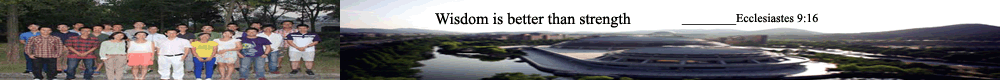Home  >  Test Objects for Computer Vision by RP

This is to briefly introduce how to make a typical object by Rapid Prototyping (RP) process, for testing of the accuracy in computer vision algorithms/methods.

Partially Funded by:
RGC Competitive Earmarked Research Grant (CERG), Hong Kong [Project 9040540]; and
Strategic Research Grant, CityU [Project 7001431].

Citations:
 S.Y. Chen and Y.F. Li, "Finding Optimal Focusing Distance and Edge Blur Distribution for Weakly Calibrated 3D Vision", IEEE Transactions on Industrial Informatics, Vol. 9, No. ?, 2013, early access.
 S.Y. Chen, Y. F. Li, J.W. Zhang, "Vision Processing for Realtime 3D Data Acquisition Based on Coded Structured Light", IEEE Transactions on Image Processing, Vol. 17, No. 2, Feb. 2008, pp. 167-176.

By S. Y. Chen and Y. F. Li, City University of Hong Kong

Contents:

Design

We define a function to generate a freeform object surface:

(1)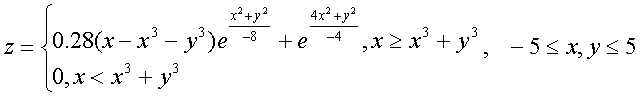or

(2)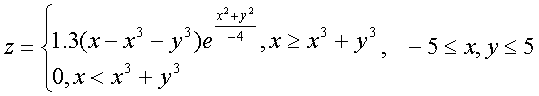Precision models

The two equations will produce model surfaces, either by AutoCAD or Matlab.

(1)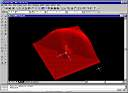or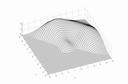(Click for full size image)
Model surface by AutoCAD or Matlab for Eq (1).

Model surface by Matlab for Eq (2).

The programs

Example one - Autolisp

The first example is to show 3D surface mesh generated by Autolisp in AutoCAD:

(defun c:fplot (/ res start x step ce)
(setq res 150
start -5.0
x start
step (/ 10.0 (float res))
i 0)
(setq ce (getvar "cmdecho"))
(setvar "cmdecho" 0)
(command "3Dmesh" res res)
(while (< i (1+ res))
(setq j 0
y start)
(while (< j res)
(setq j (1+ j)
y (+ y step))
(setq a (- (- x (expt x 3.0)) (expt y 3.0)))
(setq b (exp (/ (+ (* x x) (* y y)) -8.0)))
(setq c (exp (- (* x (* -1.0 x)) (/ (* y y) 4))))
(setq z (- (+ (* (* 0.28 a) b) c) 0.1))
(if (< z 0) (setq z 0))
(command (list x y z))
)
(setq i (1+ i)
x (+ x step))
)
(setvar "cmdecho" ce)
)

Example two - Matlab
If using Matlab, here is the scripts for Eq (2):

[X,Y] = meshgrid(-5:.2:5);
Z = 1.3*(X-X.^3-Y.^3) .* exp(-X.^2/4 - Y.^2/4);
S = Z>0;
Z=Z.*S;
mesh(X,Y,Z)
axis equal

The models produced by RP

After obtaining the 3D precision data sets, we export file formats in DXF or IGES. Then they are converted as *.STL for RP process.

Here is the model produced. It will then be used in Computer Vision, for testing of accuracy or any other purposes, because we can have both precision data sets and 3D measured data sets by vision sensors.

For more details of model reconstruction by computer vision, please read my published papers of this issue.

Program: The autolisp program for CAD, mesh3c.lsp.

3D data set: Example data of the object by Matlab (in XYZ).
Page visits:since January 1, 2000. Last updated: August 1, 2012.  sychen.com all rights reserved.